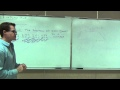# Vídeo classPrealgebra Lecture 2.1: Introduction to Integers

Prealgebra Lecture 2.1: Introduction to Integers

3.46

(48)

## 38h54m of online video course

Quality content and selected for your learning.

## Exercises to train your knowledge

Many of the courses available have exercises to help with learning.

This course is free today and always will be, from start to finish you will pay nothing.Teacher

##### Leonard
###### 6 Courses / 1571 students

This Channel is dedicated to quality mathematics education. It is absolutely FREE so Enjoy! Videos are organized in playlists and are course specific.

## Course content preview

There are no comments yet for this course.Watch the 1st video of the course

Do not worry, it is free!

## Course content0h14m

### Prealgebra Lecture 1.2: Studying Place Value and Expanded Form of Numbers

Prealgebra Lecture 1.2: Studying Place Value and Expanded Form of Numbers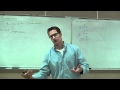0h44m

### Prealgebra Lecture 1.3: Addition and Subtraction of whole numbers. Perimeter.

Prealgebra Lecture 1.3: Addition and Subtraction of whole numbers. Perimeter.0h23m

### Prealgebra Lecture 1.4: How to do Rounding and Estimating of Whole Numbers

Prealgebra Lecture 1.4: How to do Rounding and Estimating of Whole Numbers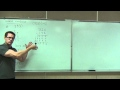0h45m

### Prealgebra Lecture 1.5: Multiplication of Whole Numbers. Area of a Rectangle

Prealgebra Lecture 1.5: Multiplication of Whole Numbers. Area of a Rectangle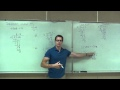0h46m

### Prealgebra Lecture 1.6: Division of Whole Numbers

Prealgebra Lecture 1.6: Division of Whole Numbers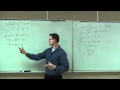1h15m

### Prealgebra Lecture 1.7: Studying Exponents and Order of Operations

Prealgebra Lecture 1.7: Studying Exponents and Order of Operations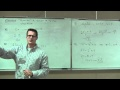1h01m

### Prealgebra Lecture 1.8: An Introduction to Variables and Algebraic Expressions

Prealgebra Lecture 1.8: An Introduction to Variables and Algebraic Expressions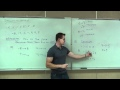0h38m

### Prealgebra Lecture 2.1: Introduction to Integers

Prealgebra Lecture 2.1: Introduction to Integers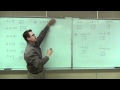0h37m

### Prealgebra Lecture 2.2: Adding of Integers. Using the Addition Rule for Integers

Prealgebra Lecture 2.2: Adding of Integers. Using the Addition Rule for Integers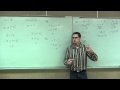0h56m

### Prealgebra Lecture 2.3: Subtracting Integers. How to Change Subtraction to Addition

Prealgebra Lecture 2.3: Subtracting Integers. How to Change Subtraction to Addition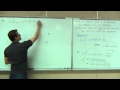0h50m

### Prealgebra Lecture 2.4: Multiplying and Dividing Integers

Prealgebra Lecture 2.4: Multiplying and Dividing Integers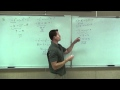2h00m

### Prealgebra Lecture 2.5: Studying Order of Operations with Integers

Prealgebra Lecture 2.5: Studying Order of Operations with Integers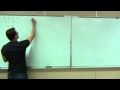1h22m

### Prealgebra Lecture 2.6: An Introduction to Solving Basic Equations

Prealgebra Lecture 2.6: An Introduction to Solving Basic Equations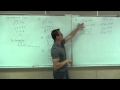2h17m

### Prealgebra Lecture 3.1: Simplifying Algebraic Expressions

Prealgebra Lecture 3.1: Simplifying Algebraic Expressions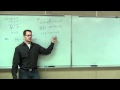1h23m

### Prealgebra Lecture 3.2: Solving Basic Equations

Prealgebra Lecture 3.2: Solving Basic Equations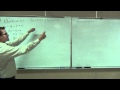1h14m

### Prealgebra Lecture 3.3: Solving General Equations. How to Solve Any Equation in Prealgebra

Prealgebra Lecture 3.3: Solving General Equations. How to Solve Any Equation in Prealgebra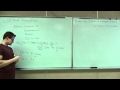0h28m

### Prealgebra Lecture 3.4: Translating Sentences Into Equations. Intro to Word Problems

Prealgebra Lecture 3.4: Translating Sentences Into Equations. Intro to Word Problems0h30m

### Prealgebra Lecture 4.1: Introduction to Fractions

Prealgebra Lecture 4.1: Introduction to Fractions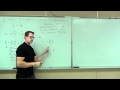1h16m

### Prealgebra Lecture 4.2: Prime Factorization and Simplification of Fractions

Prealgebra Lecture 4.2: Prime Factorization and Simplification of Fractions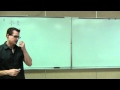1h53m

### Prealgebra Lecture 4.3: How to Multiply and Divide Fractions

Prealgebra Lecture 4.3: Multiplying and Dividing Fractions